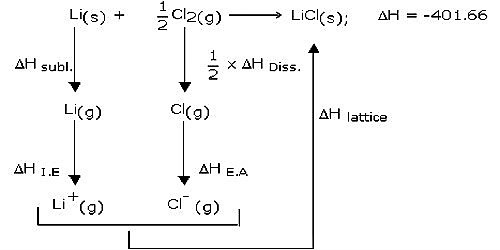# Lavoisier and Laplace’s law of Thermochemistry

Thermochemistry is the study of heat energy related to chemical reactions and/or physical transformations. Thermochemistry is helpful in predicting reactant and product quantities at all times throughout a given reaction.

Thermochemistry follows some rules. These are,

1. The amount of enthalpy transform, ΔH varies proportionally with the amount of substrate or product.
2. The amount of ΔH of a reaction is equivalent for the reverse reaction, but the sign is contradictory.
3. The magnitude of ΔH does not depend on the number of steps for a given reaction. It is similar irrespective of the number of steps for the reaction.

Lavoisier and Laplace’s law of Thermochemistry

Two laws of thermochemistry are very important in thermochemical calculations. Both laws preceded the first law of thermodynamics (1850). It will, however, be shown that the laws of Thermochemistry are a direct consequence of the first law of thermodynamics.

Lavoisier and Laplace’s law (1782): This law may be stated in the general form as the heat change accompanying a chemical reaction in one direction is exactly equal in magnitude, but opposite in sign, to that associated with the same reaction in the reverse direction. This law states that; the heat change (or enthalpy change) of a chemical reaction is exactly equal but opposite in sign for the reverse reaction.

This is evident from the following two fractions:

(a) CH4 (g) + 2O2 (g) → CO2 (g) + 2H2O (l)     [here, ∆H0 = -890.3 kJ mol-1]

(b) CO2 (g) + 2H2O (l) → CH4 (g) + 2O2 (g)      [here, ∆H0 = +890.3 kJ mol-1]

Thus, it can be concluded that ∆Hforward reaction = ∆Hbackword reaction

A chemical equation, when written along with enthalpy change for the reaction, is termed a thermochemical equation. The two equations are examples of thermochemical equations. Laplace’s law allows us to use thermochemical equations as algebraic equations.

Explanation:

Lavoisier and Laplace gave this law in 1780 which states that “the enthalpy of a reaction is accurately equal but contradictory in the sign for the reverse reaction.” It states that the heat change (or enthalpy change) of a chemical reaction is accurately equivalent but reverses in the sign for the reverse reaction.

For example, if DH is the enthalpy change in going from A to B then the enthalpy change for the process B to A would be -DH. Thus, the enthalpy of the structure of a compound is numerically equivalent but reverse in sign to the enthalpy of decomposition of the compound.

S(s) + O2(g) → SO2(g) ΔH = -296.9 kJ

SO2(g) → S(s) + O2(g) ΔH = +296.9 kJ

Whenever a thermochemical equation is reversed the sign of DH also gets reversed.

In accordance with this law enthalpy of decomposition of the compound is numerically identical to the enthalpy of the pattern of that particular compound with opposite sign,

C(s) + O2 → CO2 (g); ΔH = – 94.3 kcal

CO2 → C(s) + O2; ΔH = + 94.3 kcal.

In the Second rule of Lavoisier and Laplace can describe as, According to this rule if melting of 1 mole of ice requires enthalpy 6.4 kJ, then for the reverse process it will be -6.4 kJ. Processes with ΔH > 0 are endothermic whereas ΔH < 0 are exothermic.

Thermochemistry can explain the spontaneity and non-spontaneity of a reaction. Heat capacity, enthalpy, entropy, enthalpy of structure, enthalpy of combustion and calories can be calculated through the study of Thermochemistry.